# NCERT Solutions Class 12 Computer Science (Python) Chapter -6 (Database Concepts)

NCERT Solutions Class 12 Computer Science (Python) from class 12th Students will get the answers of Chapter-6 (Database Concepts) This chapter will help you to learn the basics and you should expect at least one question in your exam from this chapter.
We have given the answers of all the questions of NCERT Board Computer Science (Python) Textbook in very easy language, which will be very easy for the students to understand and remember so that you can pass with good marks in your examination.

Class 12 Computer Science (Python)

Chapter-6 (Database Concepts)

Questions and answers given in practice

Chapter-6 (Database Concepts)

Cartesian Product
Degree — 4
Cardinality = 6

Question 2:
Define degree and cardinality. Also, Based upon given table write degree and cardinality.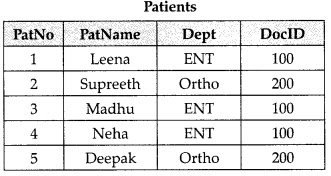Degree is the number of attributes or columns present in a table.
Cardinality is the number of tuples or rows present in a table.
Patients Degree = 4
Cardinality = 5

Question 3:
Observe the following table and answer the parts (i) and (ii):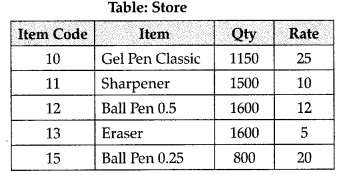1. In the above table, can we have Qty as primary key.
2. What is the cardinality and degree of the above table?

1. We cannot use Qty as primary key because there is a duplication of values and primary key value cannot be duplicate.
2. Degree =4
Cardinality = 5

Question 4:
Explain the concept of union between two tables, with the help of appropriate example.

The union operation denoted by ‘U’ combines two or more relations. The resultant of union operation contain tuples that are in either of the table or in both tables.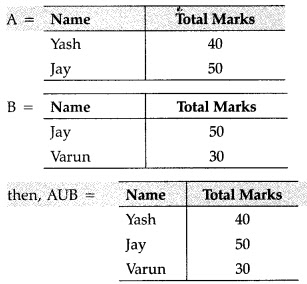Question 5:
Observe the following STUDENTS and EVENTS tables carefully and write the name of the RDBMS operation which will be used to produce the output as shown in LIST table? Also, find the degree and cardinality of the table.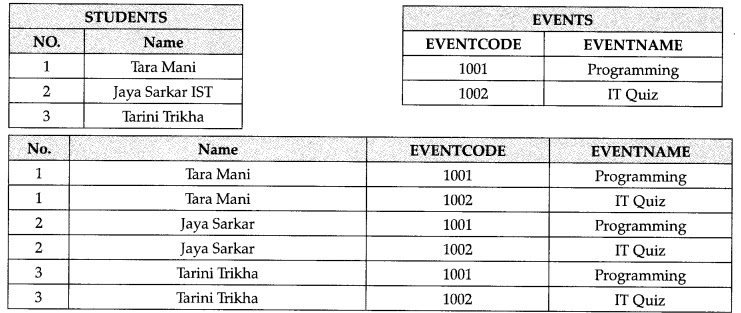Cartesian Product
Degree = 4
Cardinality = 6

Question 6:
Observe the following MEMBER and ACTIVITY tables carefully and write the name of the RDBMS operation, which will be used to produce the output as shown in REPORT? Also, find the Degree and Cardinality of the REPORT.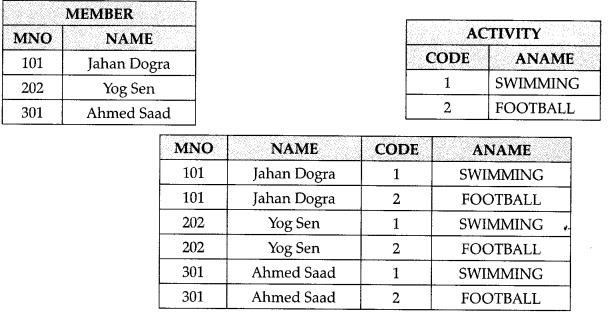Join operation or MEMBER U ACTIVITY
Degree of Report = No of columns
(No of Attributes) = 3
Candinality Report = No of Rows
(No of tuples) = 6

1. What is the cardinality and degree of the given table?
2. If a new column Contact_No has been added and three more members have joined the club then

1. Cardinality = 4 Degree = 5
2. Cardinality = 7
3. Degree = 6

Question 8:
What do you understand by Union & Cartesian product in the relational algebra?

Union of R ans S :The Union of two relations is a relation that includes all the tuples that are either in R or in S or in both R and S. Duplicate tuples are eliminated.
The Union is an operator which works on two how sets. It combines the tuples of one relation with all the tuples of the other relation such that there is no duplication.

Cartesian Product: The cartesian product is an operator which works on two sets. It combines the tuples of one relation with all the tuples of the other relation.

Question 9:
Differentiate between the Alternate key of a table with the help of an example.

Primary Key: A primary key is a value that can be used to identify a unique row in a table .
Alternate Key: An alternate key is any candidate key which is not selected to be the primary key
Example: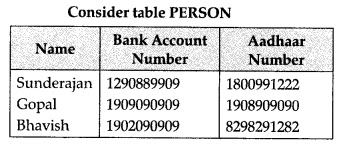So, (Bank Account Number, Aadhaar Number) are candidate keys for the table.
Bank Account Number — Alternate key

Question 10:
Explain the concept of candidate key with the help of an appropriate example.

Candidate key is a column or set of columns that can help in identifying records uniquely.
Example, consider a Table STUDENT.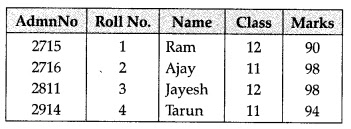Here, AdmnNo & Roll No define Table uniquely.

Question 11:
What do you understand by degree & cardinality of a Table ?

Degree refers to the number of columns in a table.
Cardinality refers to the number of rows.

1. In the above table, can we take Mno as Pri­mary key ? (Answer as [Yes/No] only.) Justify your answer with a valid reason.
2. What is the degree and the cardinality of the above table?

1. No
2. Degree = 4
Cardinality = 5
[Hint: Because Pencil and Eraser are having the same Mno = 2. Primary key needs to be unique]

Question 13:
Give a suitable example of a table with sample data and illustrate Primary and Candidate keys in it.

A table may have more than one such attribute or a group of attribute that identifies a row/ tuple uniquely, all such attribute(s) are known as Candidate keys. Out of the Candidate keys, one is selected as Primary key.Id = Primary key Id and Qty = Candidates Keys

Question 14:
What do you understand by selection and projection operations in the relational algebra?

Projection (n): In relational algebra, projection is a unary operation. The result of such projection is defined as the set obtained when the com­ponents of the tuple R are restricted to the set {a1…,an} – it discards (or exculdes) the other attributes.
Selection (σ): In relational algebra, a selection is a unary operation written as raib (R) or vaiv(R) where:

1. a and b are attribute names
2. i is a binary operation in the set
3. v is a value constant
4. R is a relation

The selection vσ(R) selects all those tuples in R for which i holds between the a atribute and the b attribute.

Question 15:
What do you understand by Primary key and Candidate keys.

An attribute or set of attributes which are used to identify a tuple uniquely is known as primary key. If a tuple has more than one such attribute which identify a tuple uniquely, than all such attributes are known as candidate keys.

Question 16:
What is relation? Define the relational data model.

A relation is a table having atomic values, unique row, and unordered rows and columns. The relational model represent data and relationship among data by a collection of tables known as relation, each of which has a number of columns with unique names.

Question 17:
Define domain with respect to database. Give an example.

A domain is a pool of values from which the actual values appearing in a given column are drawn.
For example: The values appearing in the Supp# column of both the suppliers table and the Shipment table are drawn from the same domain.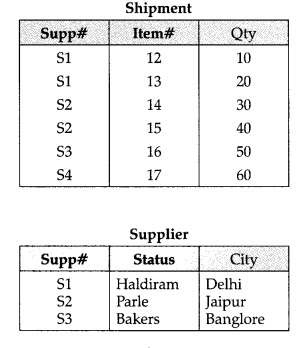Question 18:
Expand the following:

1. SQL
2. DBMS

1. SQL – Structured Query Language.
2. DBMS – Data Base Management System.

Question 19:
What do you understand by candidate keys in a table? Give a suitable example of candidate keys from a table containing some meaningful data.

Candidate key: A candidate key is one that can identify each row of a table uniquely. Generally, a candidate key becomes the primary key of the table. If the table has more than one candidate key, one of them will become the primary key, and the rest are called alternate keys.
Example: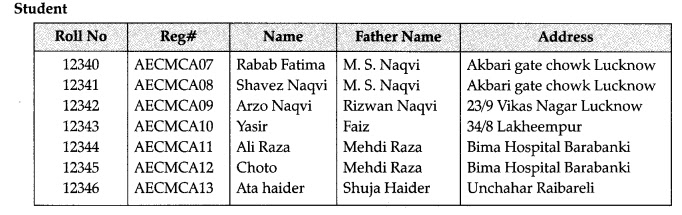Question 20:
What are all the domain names possible in gen­der ?

Male and Female

Question 21:
A table ‘customer’ has 10 columns but no row. Later, 10 new rows are inserted and 3 rows are deleted in the table. What is the degree and cardinality of the table customer.

Degree = 10 [no. of cols]
Cardinality = 10-3 = 7 [no. of rows]

Question 22:
A table ‘student’ has 3 columns and 10 rows and another table ‘student 2’ has the same columns as student but 15 rows. 5 rows are common in both the tables. If we take union, what is the degree and cardinality of the resultant table ?

Degree = 3
Cardinality = 30 (20 + 15 – 5)

Question 23:
A table ‘student’ has 4 columns and 10 rows and ‘student 2’ has 5 columns and 5 rows. If we take cartesian product of these two tables, what is the degree and cardinality of the resultant table ?

Degree = 4 x 5 = 20 [no. of columns]
Cardinality = 10 x 5 = 50 [no. of rows]